# 房地产周期性波动的谱分析研究Study on Spectral Analysis of Real Estate Cyclic Fluctuation

DOI: 10.12677/SA.2018.72026, PDF, HTML, XML, 下载: 843  浏览: 3,021

Abstract: This paper first briefly describes the theoretical tools on studying economic data with periodic characteristics, then key indicators both of the supply and demand are identified by extracting the principal components from statistical data of real estate market in China from 1988 to 2016. Discussing the stability characteristics of the key indicators, the cycles both of the supply and demand is calculated by using spectral analysis techniques. The results show that both supply and demand fluctuate periodically. The supply has a main cycle about 3.5-year long and a secondary 5.6-year cycle. In the meantime, the demand has a main cycle of 4.67 and a sub-cycle of 2.8 years. From the long-term development, the supply and demand would have the trend of balanced development.

1. 引言

2. 数据处理的理论基础

2.1. 主成分分析法

${\beta }_{1}\triangleq {\alpha }_{1}^{\text{T}}x={\alpha }_{11}{x}_{1}+{\alpha }_{12}{x}_{2}+\cdots +{\alpha }_{1n}{x}_{n}$

s.t. $\mathrm{var}\left({\beta }_{1}\right)$ 最大，且 ${\alpha }_{1}^{\text{T}}\cdot {\alpha }_{1}=1$

${\beta }_{2}\triangleq {\alpha }_{2}^{\text{T}}x={\alpha }_{21}{x}_{1}+{\alpha }_{22}{x}_{2}+\cdots +{\alpha }_{2n}{x}_{n}$

s.t. $\mathrm{var}\left({\beta }_{2}\right)$ 最大，且 ${\alpha }_{2}^{\text{T}}\cdot {\alpha }_{2}=1$${\beta }_{1}$${\beta }_{2}$ 线性无关

2.2. 谱分析方法

${X}_{t}={A}_{0}+\underset{m=1}{\overset{n}{\sum }}\left({A}_{m}\mathrm{cos}\left(2\text{π}mt/N\right)+{B}_{m}\mathrm{sin}\left(2\text{π}mt/N\right)\right)+{\epsilon }_{t},\text{\hspace{0.17em}}\text{\hspace{0.17em}}t=1,2,\cdots ,N$ , (1)

$\begin{array}{c}{X}_{t}={A}_{0}+\underset{m=1}{\overset{n}{\sum }}\left\{\left(\frac{2}{N}\underset{t=1}{\overset{N}{\sum }}{X}_{t}\mathrm{cos}\left(2\text{π}mt/N\right)\right)\mathrm{cos}\left(2\text{π}mt/N\right)\\ \text{\hspace{0.17em}}\text{\hspace{0.17em}}+\left(\frac{2}{N}\underset{t=1}{\overset{N}{\sum }}{X}_{t}\mathrm{sin}\left(2\text{π}mt/N\right)\right)\mathrm{sin}\left(2\text{π}mt/N\right)\right\}+{\epsilon }_{t}\\ ={A}_{0}+2\underset{m=1}{\overset{n}{\sum }}\left\{\left(\frac{1}{N}\underset{t=1}{\overset{N}{\sum }}{X}_{t}\mathrm{cos}\left(2\text{π}mt/N\right)\right)\mathrm{cos}\left(2\text{π}mt/N\right)\\ \text{\hspace{0.17em}}\text{\hspace{0.17em}}+\left(\frac{1}{N}\underset{t=1}{\overset{N}{\sum }}{X}_{t}\mathrm{sin}\left(2\text{π}mt/N\right)\right)\mathrm{sin}\left(2\text{π}mt/N\right)\right\}+{\epsilon }_{t}\end{array}$

${\stackrel{¯}{A}}_{m}=\frac{1}{N}\underset{t=1}{\overset{N}{\sum }}{X}_{t}\mathrm{cos}\left(2\text{π}mt/N\right),\text{}m=1,2,\cdots ,n$ (2)

${\stackrel{¯}{B}}_{m}=\frac{1}{N}\underset{t=1}{\overset{N}{\sum }}{X}_{t}\mathrm{sin}\left(2\text{π}mt/N\right),\text{}m=1,2,\cdots ,n$ (3)

$I\left({f}_{m}\right)=N\left({\stackrel{¯}{A}}_{m}^{2}+{\stackrel{¯}{B}}_{m}^{2}\right),\text{}m=1,2,\cdots ,n$ (4)

$周期长度=\mathrm{max}\left\{N\left({\stackrel{¯}{A}}_{1}^{2}+{\stackrel{¯}{B}}_{1}^{2}\right)\to N,N\left({\stackrel{¯}{A}}_{2}^{2}+{\stackrel{¯}{B}}_{2}^{2}\right)\to \frac{N}{2},\cdots ,N\left({\stackrel{¯}{A}}_{n}^{2}+{\stackrel{¯}{B}}_{n}^{2}\right)\to \frac{N}{n}\right\}$ .

3. 实证分析研究

3.1. 供给类与需求类指标选择

3.2. 供需双方数据及其规范化Table 1. Variables of both suppliers and demandersTable 2. Data of both supply and demand in real estate industry (1987-2016)Table 3. Standardized data of both supply and demand in real estate industry (1988-2016)

3.3. 主成分提取Table 4. From the Supply data: Eigenvalues and the corresponding principal componentsTable 5. From the Demand data: Eigenvalues and the corresponding principal components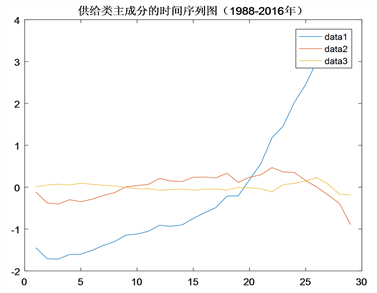Figure 1. Time-series graphs of the supplies’ principal components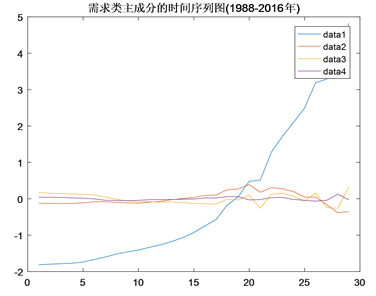Figure 2. Time-series graphs of the demands’ principal components

3.4. 频率谱分析Table 6. Synthetical variable information of supply and demand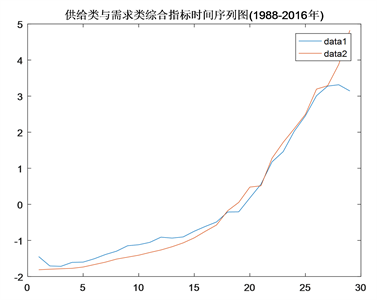Figure 3. Time-series graph of the synthetical viables of supply and demand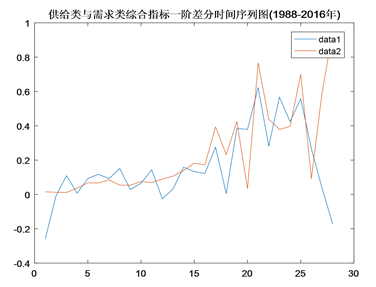Figure 4. First-order difference time-series graph of the synthetical viables of supply and demand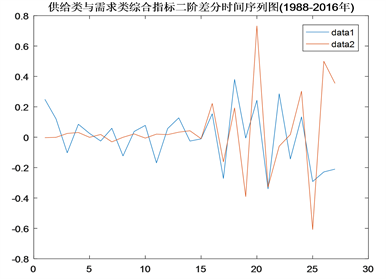Figure 5. Second-order difference time-series graph of the synthetical viables of supply and demand

$yy=0.8481\ast xx,\text{}且\text{bint}=\left[0.\text{7997},0.\text{8965}\right],$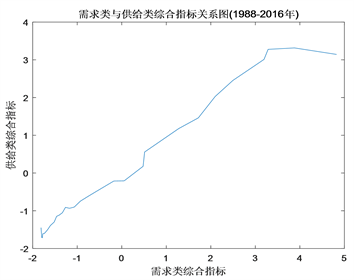Figure 6. Relation of the synthetical viables of supply and demand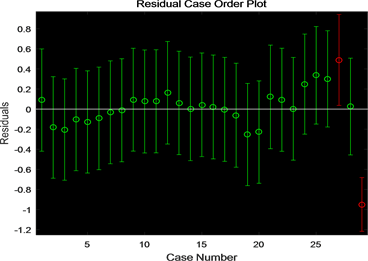Figure 7. Residual case order plot on the relation of the synthetical viables of supply and demand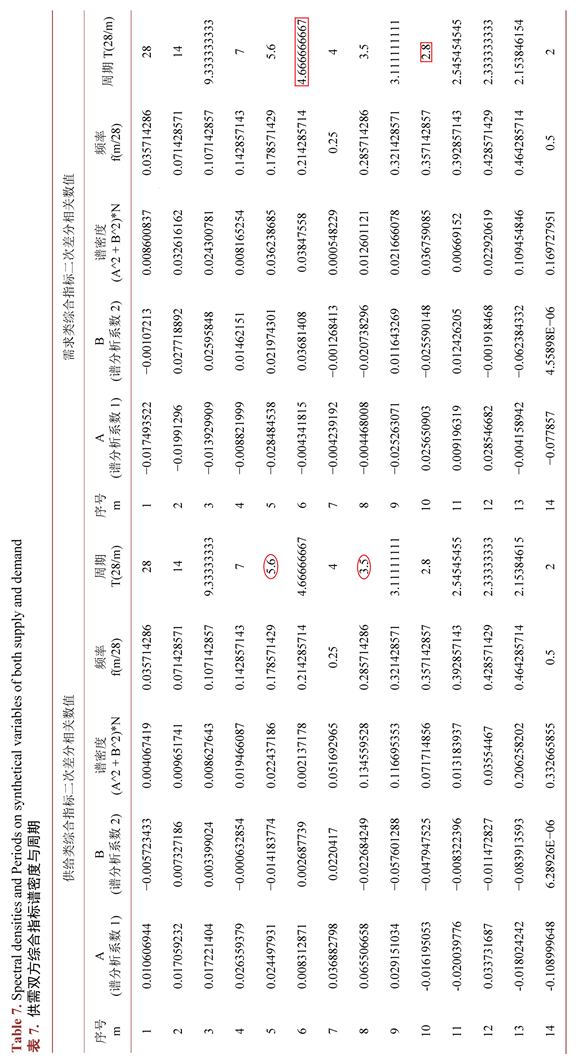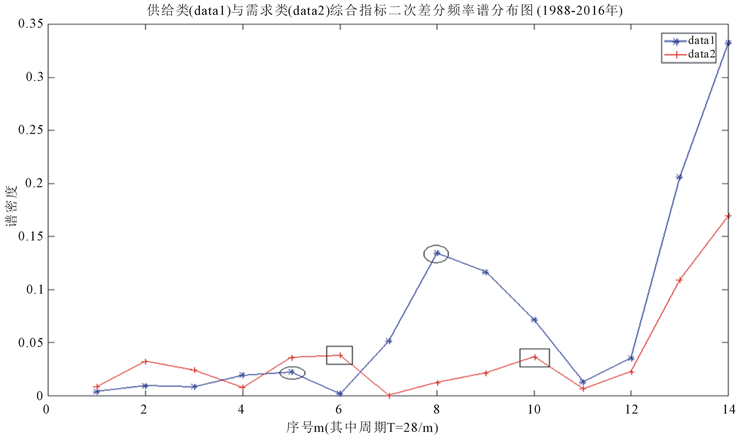Figure 8. Second-order difference Spectral density Plot of synthetical viables on supply and demand

3.5. 周期性说明

4. 结论

1988~2016年全国房地产市场的供给类与需求类均具有明显的短周期的周期特征。我们给出的周期与张红  、李玉梅  等得出的主周期或次周期不完全一致，但相差不大，说明全国性房地产行业的供需周期与地方性房地产行业供需情况或价格波动联系比较紧密，我国1988~2016年房地产行业周期与1998~2010年我国经济主周期2.23也基本吻合。

NOTES

1这里的x已经标准化，即已中心化、均值化。

2尽管主成分分析没有忽略协方差和相关性，但是更注重方差。

  Wernecke, M., Rottke, N. and Holzmann, C. (2004) Incorporating the Real Estate Cycle into Management Decisions Evidence from Germany. Real Estate Port Folio Management, 10, 171-186.  William, W.C. (1999) Real Estate Cycle: Some Funda-mentals. Real Estate Economics, 27, 209-230. https://doi.org/10.1111/1540-6229.00772  Kydland, F.E. and Prescott, E.C. (1982) Time to Build and Aggregate Fluctuations. Econometrica, 6, 1345-1370. https://doi.org/10.2307/1913386  Nelson, C.R. and Plosser, C.I. (1982) Trends and Random Walks in Macroeconomic Time Series. Journal of Monetary Economics, 2, 139-162. https://doi.org/10.1016/0304-3932(82)90012-5  范小云, 袁梦怡, 肖立晟. 理解中国的金融周期: 理论、测算与分析[J]. 国际金融研究, 2017(1): 28-38.  熊彼特. 经济发展理论(中译本) [M]. 北京: 商务印书馆, 1990.  马凤娣. 熊彼特的经济发展理论[J]. 学术论坛, 1999(1): 23-27.  Priestley, M.B. (1981) Spectral Analysis and Time Series. Academic Press, London.  Hotelling, H. (1933) Analysis of a Complex of Statis-tical Variables into Principal Components. Education Psychology, 24, 417-444.  曾峣. 经济周期与谱分析研究[J]. 贵州财经学院学报, 1999(3): 43-46.  张红, 谢娜. 基于主成分分析与谱分析的房地产市场周期研究[J]. 清华大学学报(自然科学版), 2008, 48(9): 24-27.  陈磊, 张屹山. 我国转轨时期经济周期波动的谱分析[ J]. 数量经济技术经济研究, 2001(1): 18-21.  李玉梅. 我国房地产价格变动特征及其影响因素的实证研究[D]: [博士学位论文]. 吉林: 吉林大学, 2012.  王悦. 谱分析方法及其在经济周期研究中的应用谱分析方法及其在经济周期研究中的应用——以美国经济周期波动(1930~2009)的谱分析为例[J]. 财经科学, 2011, 284(11): 34-43.  邬琼. 谱分析方法在研究我国经济周期中的应用[J]. 中国物价, 2017(7): 3-6.  Massy, W.F. (1965) Principal Components Regression in Exploratory Statistical Research. Journal of the American Statistical Association, 60, 234-256. https://doi.org/10.1080/01621459.1965.10480787